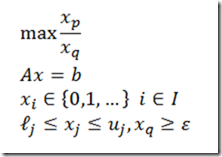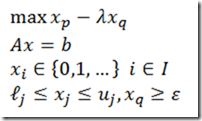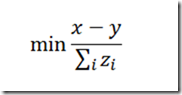## Monday, January 30, 2012

### Formal proof?

Is there an easy formal proof for this: http://yetanothermathprogrammingconsultant.blogspot.com/2011/10/special-case-of-integer-cuts.html? I think I derived it once. The more general cut is derived here: http://yetanothermathprogrammingconsultant.blogspot.com/2011/10/integer-cuts.html.

Update. Here are my dabblings. First we go back to the derivation shown here and take the final formulation for the cut. From there we can do:## Monday, January 23, 2012

### Comparing some modeling languages

Not an easy task, as can be seen here:

http://lists.gnu.org/archive/html/help-glpk/2012-01/msg00033.html

To really appreciate the features of a modeling system requires quite some investment. When comparing more systems, also often a “least common denominator” model is used. Some issues are more specialized, such as:

1. Is there support for non-linear programming
2. Performance characteristics for very large models
3. Ability to do data manipulation efficiently
4. Ability to write tailored algorithms if the model is not amenable to standard solution methods (see e.g. http://yetanothermathprogrammingconsultant.blogspot.com/2012/01/dinkelbachs-algorithm.html)
5. Ability to debug large models and view large data sets

Some of these points are more difficult to evaluate and to weigh in a comparison.

PS: see comment below for some other interesting capabilities.

## Saturday, January 21, 2012

### network

I have a question: In GAMS, is there something similar to "structure" in C ?
I need to define a network optimization problem and I need to define: A node as the structure, incoming arcs as one attribute, and outgoing arcs as another attribute.

GAMS does not have that and most likely you don’t “need” that anyway. A compact way to describe a network is shown here:

http://yetanothermathprogrammingconsultant.blogspot.com/2011/06/network-formulation.html

Some small improvements over this formulation for even larger problems are documented here: http://yetanothermathprogrammingconsultant.blogspot.com/2011/08/more-on-network-models.html.

## Monday, January 16, 2012

### Dinkelbach’s Algorithm

The paper:

Dinkelbach’s Algorithm as an Efficient Method for Solving a Class of
MINLP Models for Large-Scale Cyclic Scheduling Problems  [link]

by Prof. Grossmann e.a. investigates some mixed-integer linear fractional programming problems. It compares some standard MINLP codes against an implementation of Dinkelbach’s algorithm and concludes that this algorithm performs very good. The algorithm consists of a solving a series of MIP problems with each time a different weighted objective function. This method is so simple it can be coded in GAMS in just a few lines.

If the problem is something like:then we need to solve a series of MIP problems:for different values of λ.

I wanted to see if I could use this on a (very) large mixed-integer linear fractional programming problem. Of course first try it on some very small examples. The first test is a continuous problem:

### Example 1: Linear fractional programming problem

 \$ontext    References:      Fengqi You, Pedro M. Castro, Ignacio E. Grossmann1      Dinkelbach’s Algorithm as an Efficient Method for Solving a Class of      MINLP Models for Large-Scale Cyclic Scheduling Problems      Said Tantawy      AN ITERATIVE METHOD FOR SOLVING LINEAR FRACTION      PROGRAMMING (LFP) PROBLEM WITH SENSITIVITY ANALYSIS \$offtext set    i 'products' /a1,a2/ ; parameters    uprofit(i) 'unit profit' /       a1 4       a2 2    /    ucost(i) 'unit cost' /       a1 1       a2 2    /    fixedcost /5/    fixedprofit /10/    matuse(i) 'raw material usage' /       a1 1       a2 3    /    rawavail 'raw material available' /30/ ; variables    x(i) 'production'    profit    cost    z    'objective variable' ; positive variables x,profit,cost; equations    eprofit 'calculate profit'    ecost   'calculate cost'    eraw    'raw material usage'    eprodcon  'production constraint'    eratio   'minlp objective' ; eprofit.. profit =e= fixedprofit + sum(i, uprofit(i)*x(i)); ecost..   cost =e= fixedcost + sum(i, ucost(i)*x(i)); eraw..    sum(i, matuse(i)*x(i)) =l= rawavail; eprodcon..  x('a1') + 5 =g= 2*x('a2'); eratio..   z =e= profit/cost; cost.lo = 1; *------------------------------------------------------------- * solve as nlp *------------------------------------------------------------- model m1 /all/; m1.solprint = 2; solve m1 maximizing z using nlp; display "------------------------------------",         "NLP Solver",         "------------------------------------",         z.l,x.l; *------------------------------------------------------------- * Dinkelbach's algorithm *------------------------------------------------------------- scalars   q  'optimal objective at end of algorithm' /0/   continue /1/   tol  /0.1/   iterations ; equation linobj; linobj .. z =e= profit - q*cost; model m2 /eprofit,ecost,eraw,eprodcon,linobj/; m2.solprint = 2; set iter /iter1*iter5/; loop(iter\$continue,    solve m2 maximizing z using lp;    if (z.l < tol,       continue = 0;       iterations = ord(iter);       display "------------------------------------",               "Dinkelbach algorithm",               "------------------------------------",               q,iterations,x.l;    else       q = profit.l/cost.l;    ); );

This continuous problem is very easy:

 ----     73 ------------------------------------             NLP Solver             ------------------------------------             VARIABLE z.L                   =        3.714  objective variable ----     73 VARIABLE x.L  production a1 30.000 ----    102 ------------------------------------             Dinkelbach algorithm             ------------------------------------             PARAMETER q                    =        3.714  optimal objective at end of algorithm             PARAMETER iterations           =            2  ----    102 VARIABLE x.L  production a1 30.000

The next small example is an integer problem:

### Example 2: an integer fractional programming problem

 \$ontext    References:      Fengqi You, Pedro M. Castro, Ignacio E. Grossmann1      Dinkelbach’s Algorithm as an Efficient Method for Solving a Class of      MINLP Models for Large-Scale Cyclic Scheduling Problems      Ildiko Zsigmond      Mixed Integer linear Fractional Programming By A Branch-and-Bound      Technique 1985 \$offtext variables    x1,x2    num  'numerator'    denom  'denominator'    z    'objective variable' ; integer variables x1,x2; x1.up = 5; x2.up = 4; equations    enum    'numerator'    edenom   'denominator'    e1,e2,e3    eratio ; enum.. num =e= 2*x1+x2-2; edenom..  denom =e= x1-x2+1; e1.. -5*x1 + 4*x2 =l= 0; e2.. -x1+x2 =l= 0.5; e3.. 2*x1+x2 =l= 11; eratio..   z =e= num/denom; denom.lo = .1; option optcr=0; model m1 /enum,edenom,e1,e2,e3,eratio/; m1.solprint = 2; solve m1 maximizing z using minlp; display "------------------------------------",         "MINLP Solver",         "------------------------------------",         z.l,x1.l,x2.l; *------------------------------------------------------------- * Dinkelbach's algorithm *------------------------------------------------------------- scalars   q  'optimal objective at end of algorithm' /0/   continue /1/   tol  /0.1/   iterations ; equation linobj; linobj .. z =e= num - q*denom; model m2 /enum,edenom,e1,e2,e3,linobj/; m2.solprint = 2; set iter /iter1*iter5/; display "------------------------------------"; loop(iter\$continue,    solve m2 maximizing z using mip;    if (z.l < tol,       continue = 0;       iterations = ord(iter);       display "------------------------------------",               "Dinkelbach algorithm",               "------------------------------------",               q,iterations,x1.l,x2.l;    else       q = num.l/denom.l;    ); );

The listing file shows:

 ----     51 ------------------------------------             MINLP Solver             ------------------------------------             VARIABLE z.L                   =        7.000  objective variable             VARIABLE x1.L                  =        3.000              VARIABLE x2.L                  =        3.000  ----     82 ------------------------------------             Dinkelbach algorithm             ------------------------------------             PARAMETER q                    =        7.000  optimal objective at end of algorithm             PARAMETER iterations           =        4.000              VARIABLE x1.L                  =        3.000              VARIABLE x2.L                  =        3.000

For our very large problem (approx. 1 million rows) this method was even more successful. All NLP solvers I have access to had troubles with the sheer size of the problem, even though the NLP relaxations are linearly constrained. But the MIP problems generated inside Dinkelbach’s algorithm turned out to be large but easy to solve. In addition the algorithm converged in about 5 major iterations.

## Tuesday, January 10, 2012

### Reviewing NLP model

In a fairly complex model, we ended up trying a nonlinear objective:where the rest of the model was linear (after applying some reformulations). As the model contains both integer and binary variables, this now has become an MINLP.

For several reasons this is actually not a very good expression:

• this expression generates a lot of non-linear variables and non-linear non-zero elements in the matrix
• as some z(i)’s can be zero it is difficult to protect this against division-by-zero (although the way we ran it had a linear model in front of this model, so our starting point was reasonable and no division-by-zero occurred)

A better formulation would be:Here we only have two non-linear variables: the rest of the variables is now appearing linearly in the constraints. In addition, although we cannot bound each z(i) away from zero, we could introduce a nonzero lower bound on w.

Although we are adding extra constraints and variables to the MINLP model, this actually makes the model easier to solve and more robust.

PS1. The model is now becoming somewhat complex as we try to deal with different types of decisions in the same model. We call this an “integrated model” rather than just messy!

PS2. In some cases fractions can be reformulated using a “fractional programming” technique. In practice for larger models this often turns out to be a difficult reformulation to implement.

Question: it is suggested in a comment below to use (2d?) bisection. Is that really a good idea? Any references for such an approach applied in a similar situation?